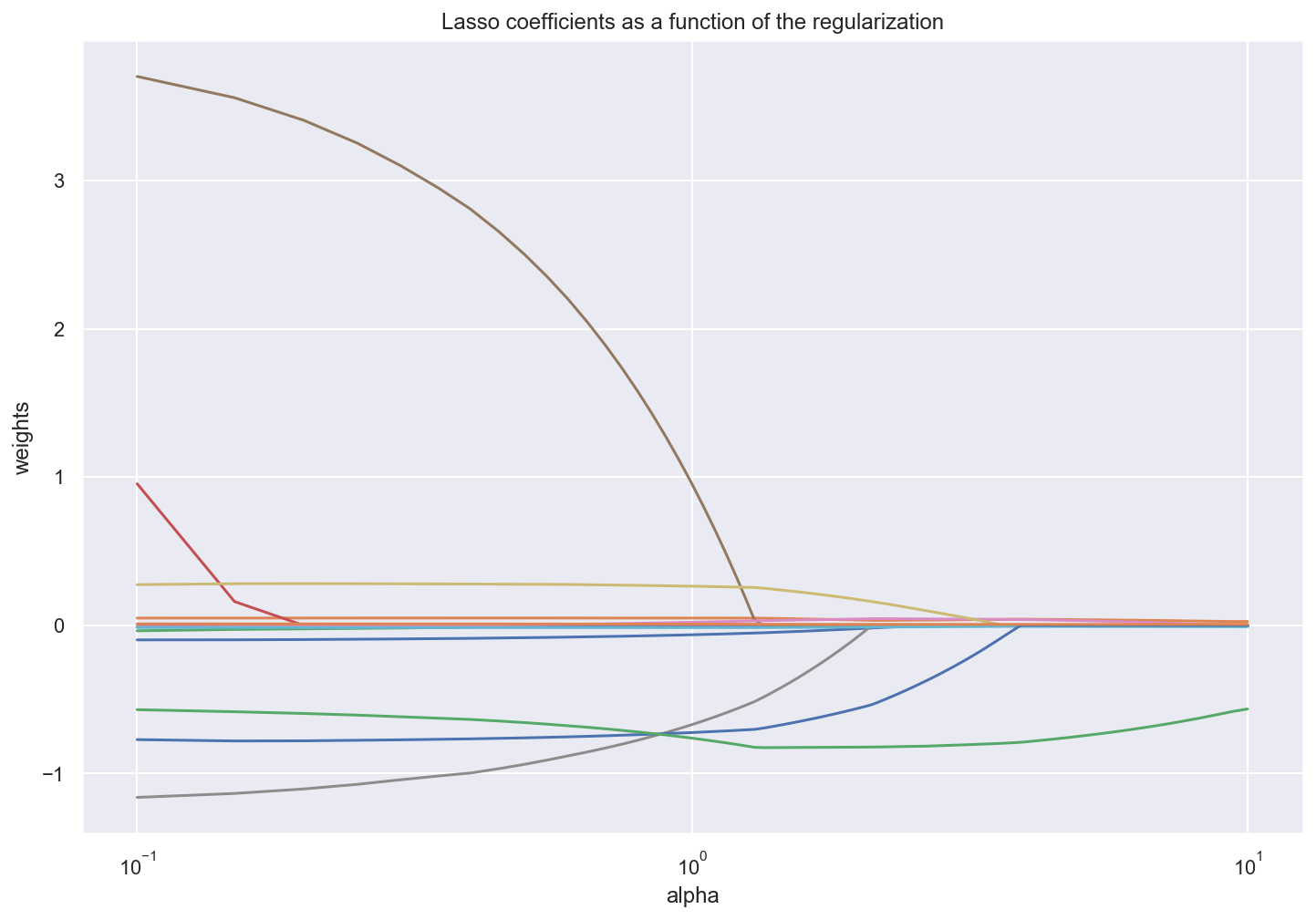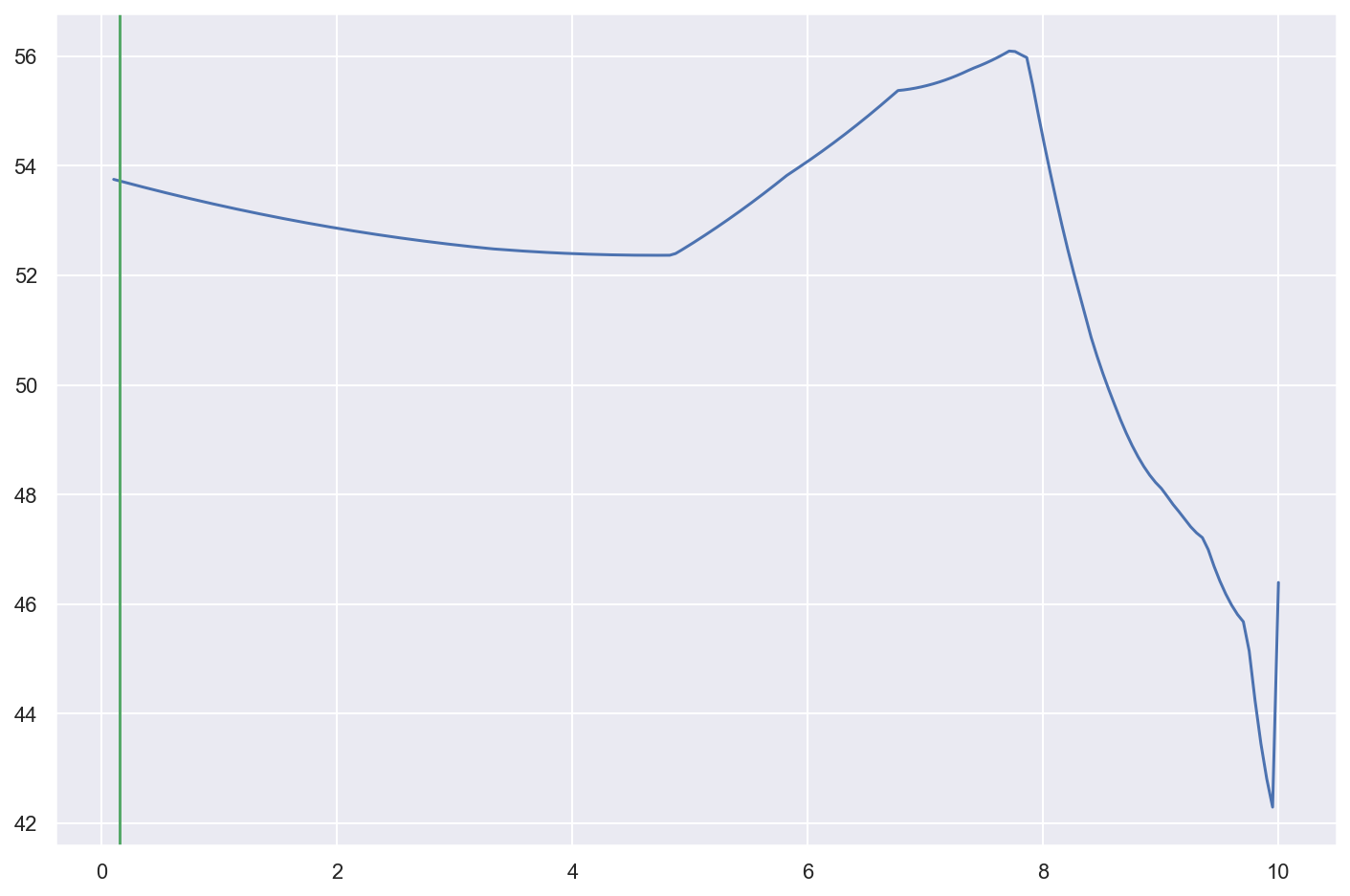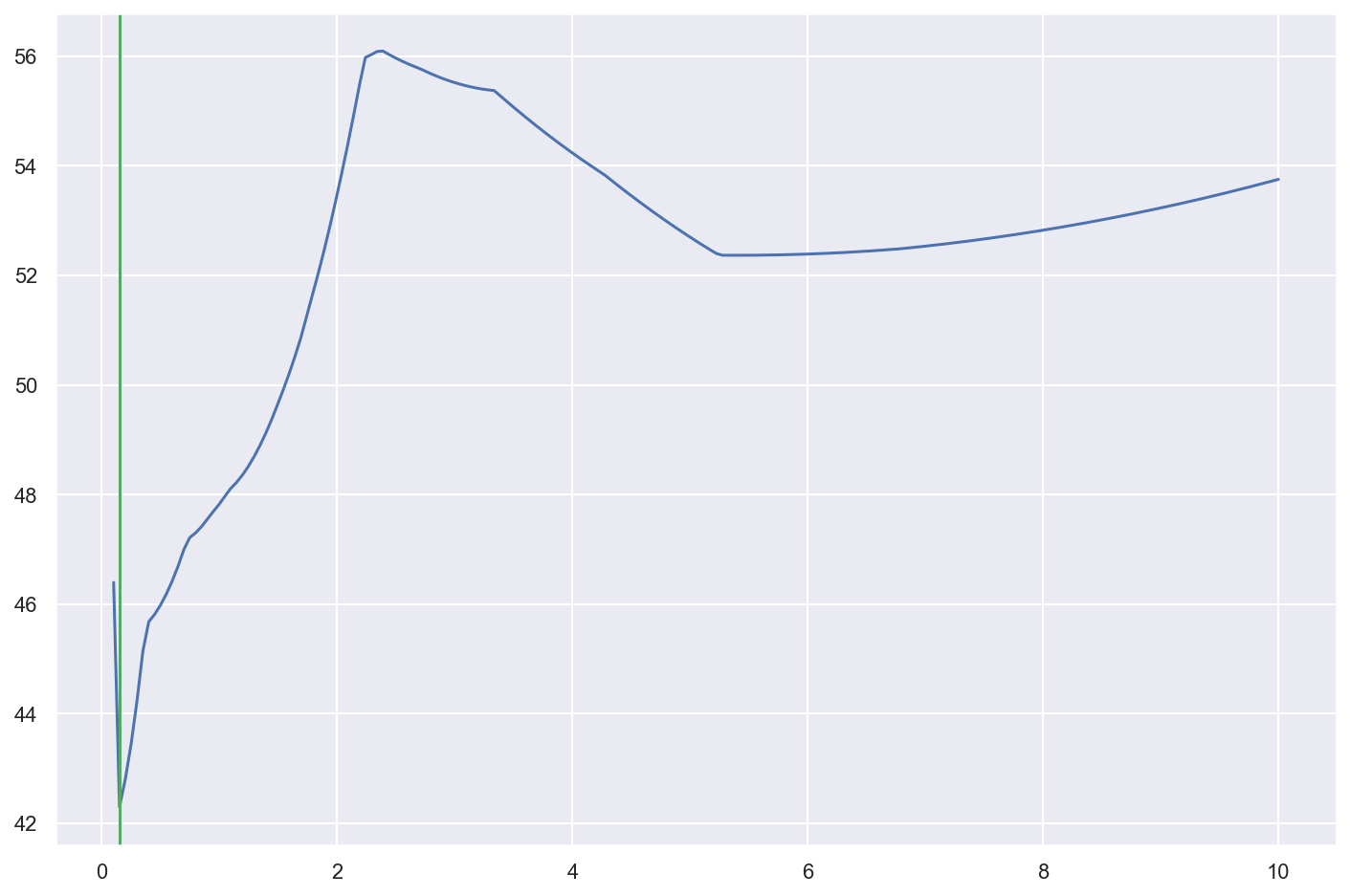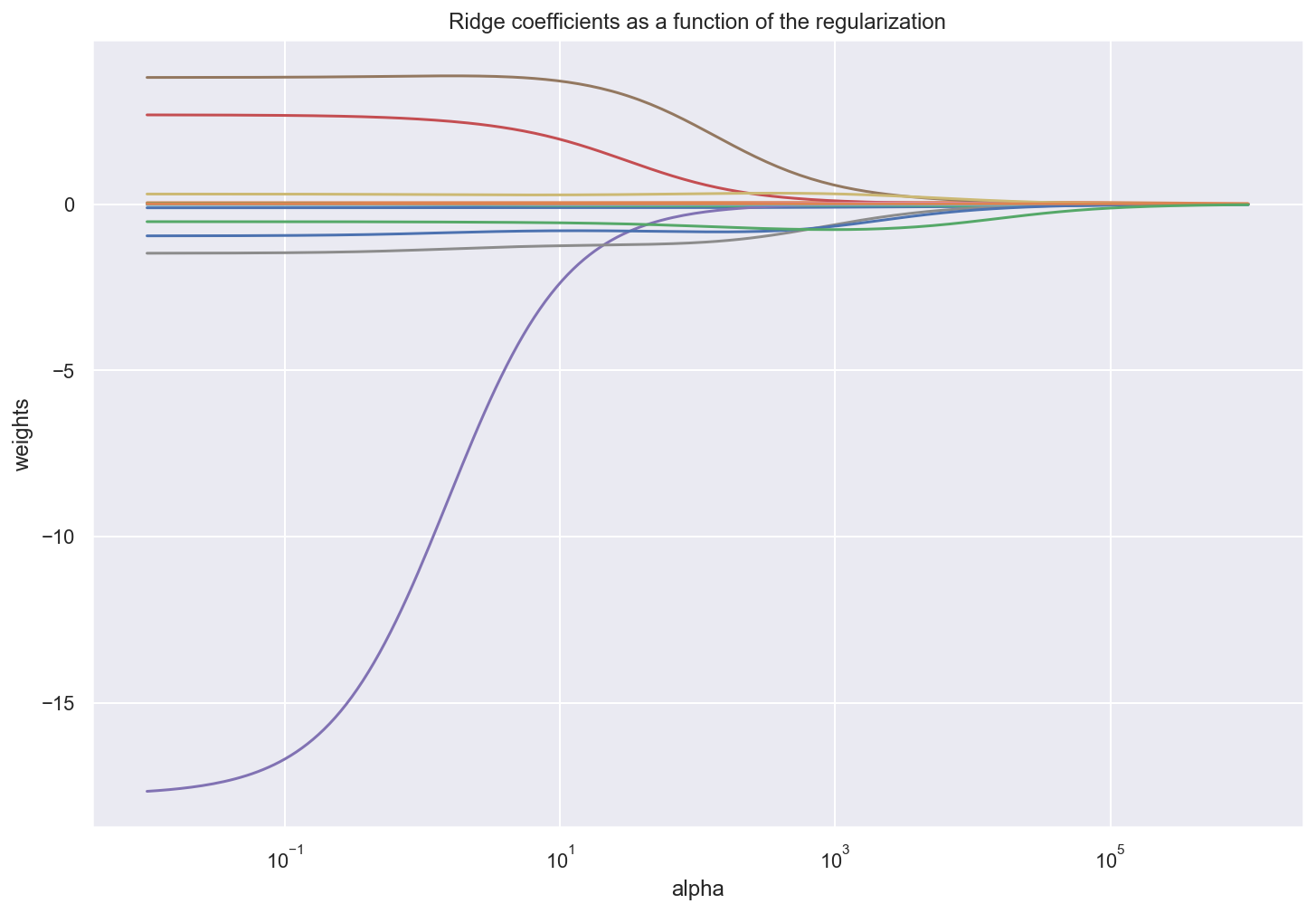## mlcourse.ai – Open Machine Learning Course¶

Author: Yury Kashnitskiy. Translated by Anna Larionova. This material is subject to the terms and conditions of the Creative Commons CC BY-NC-SA 4.0 license. Free use is permitted for any non-commercial purpose.

# Topic 6. Regression¶

## Lasso and Ridge Regressions¶

Lecture syllabus differs this week from the article outline, because topic 4 (linear models) is too huge and important, so we cover regression this week.

In :
import numpy as np
import pandas as pd

from matplotlib import pyplot as plt
%config InlineBackend.figure_format = 'retina'
import seaborn as sns; sns.set() # just to use the seaborn theme

from sklearn.model_selection import cross_val_score, KFold
from sklearn.linear_model import LassoCV, RidgeCV, Lasso, Ridge

//anaconda3/lib/python3.7/site-packages/statsmodels/tools/_testing.py:19: FutureWarning: pandas.util.testing is deprecated. Use the functions in the public API at pandas.testing instead.
import pandas.util.testing as tm


We will work with Boston house prices data (UCI repository). Download the data.

In :
boston = load_boston()
X, y = boston['data'], boston['target']


In :
print(boston.DESCR)

.. _boston_dataset:

Boston house prices dataset
---------------------------

**Data Set Characteristics:**

:Number of Instances: 506

:Number of Attributes: 13 numeric/categorical predictive. Median Value (attribute 14) is usually the target.

:Attribute Information (in order):
- CRIM     per capita crime rate by town
- ZN       proportion of residential land zoned for lots over 25,000 sq.ft.
- INDUS    proportion of non-retail business acres per town
- CHAS     Charles River dummy variable (= 1 if tract bounds river; 0 otherwise)
- NOX      nitric oxides concentration (parts per 10 million)
- RM       average number of rooms per dwelling
- AGE      proportion of owner-occupied units built prior to 1940
- DIS      weighted distances to five Boston employment centres
- TAX      full-value property-tax rate per $10,000 - PTRATIO pupil-teacher ratio by town - B 1000(Bk - 0.63)^2 where Bk is the proportion of blacks by town - LSTAT % lower status of the population - MEDV Median value of owner-occupied homes in$1000's

:Missing Attribute Values: None

:Creator: Harrison, D. and Rubinfeld, D.L.

This is a copy of UCI ML housing dataset.
https://archive.ics.uci.edu/ml/machine-learning-databases/housing/

This dataset was taken from the StatLib library which is maintained at Carnegie Mellon University.

The Boston house-price data of Harrison, D. and Rubinfeld, D.L. 'Hedonic
prices and the demand for clean air', J. Environ. Economics & Management,
vol.5, 81-102, 1978.   Used in Belsley, Kuh & Welsch, 'Regression diagnostics
...', Wiley, 1980.   N.B. Various transformations are used in the table on
pages 244-261 of the latter.

The Boston house-price data has been used in many machine learning papers that address regression
problems.

.. topic:: References

- Belsley, Kuh & Welsch, 'Regression diagnostics: Identifying Influential Data and Sources of Collinearity', Wiley, 1980. 244-261.
- Quinlan,R. (1993). Combining Instance-Based and Model-Based Learning. In Proceedings on the Tenth International Conference of Machine Learning, 236-243, University of Massachusetts, Amherst. Morgan Kaufmann.


In :
boston.feature_names

Out:
array(['CRIM', 'ZN', 'INDUS', 'CHAS', 'NOX', 'RM', 'AGE', 'DIS', 'RAD',
'TAX', 'PTRATIO', 'B', 'LSTAT'], dtype='<U7')

Let's look at the first two records.

In :
X[:2]

Out:
array([[6.3200e-03, 1.8000e+01, 2.3100e+00, 0.0000e+00, 5.3800e-01,
6.5750e+00, 6.5200e+01, 4.0900e+00, 1.0000e+00, 2.9600e+02,
1.5300e+01, 3.9690e+02, 4.9800e+00],
[2.7310e-02, 0.0000e+00, 7.0700e+00, 0.0000e+00, 4.6900e-01,
6.4210e+00, 7.8900e+01, 4.9671e+00, 2.0000e+00, 2.4200e+02,
1.7800e+01, 3.9690e+02, 9.1400e+00]])

## Lasso Regression¶

Lasso regression minimizes mean squared error with L1 regularization: $$\Large error(X, y, w) = \frac{1}{2} \sum_{i=1}^\ell {(y_i - w^Tx_i)}^2 + \alpha \sum_{i=1}^d |w_i|$$

where $y = w^Tx$ hyperplane equation depending on model parameters $w$, $\ell$ is number of observations in data $X$, $d$ is number of features, $y$ target values, $\alpha$ regularization coefficient.

Let's fit Lasso Regression with the small $\alpha$ coefficient (weak regularization). Coefficient related to NOX feature (nitric oxides concentration) will be zero. It means that this feature is the least important for median house prices prediction in this region.

In :
lasso = Lasso(alpha=0.1)
lasso.fit(X,y)
lasso.coef_

Out:
array([-0.09789363,  0.04921111, -0.03661906,  0.95519003, -0.        ,
3.70320175, -0.01003698, -1.16053834,  0.27470721, -0.01457017,
-0.77065434,  0.01024917, -0.56876914])

Let's train Lasso Regression with $\alpha=10$. All of the coefficients are equal to zero except features ZN (proportion of residential land zoned for lots over 25,000 sq.ft.), TAX (full-value property-tax rate), B (proportion of blacks by town) and LSTAT (% of lower status of the population).

In :
lasso = Lasso(alpha=10)
lasso.fit(X,y)
lasso.coef_

Out:
array([-0.        ,  0.02614602, -0.        ,  0.        ,  0.        ,
0.        ,  0.        , -0.        ,  0.        , -0.00928242,
-0.        ,  0.00749584, -0.56403807])

It means that Lasso Regression may serve as a feature selection method.

In :
n_alphas = 200
alphas = np.linspace(0.1, 10, n_alphas)
model = Lasso()

coefs = []
for a in alphas:
model.set_params(alpha=a)
model.fit(X, y)
coefs.append(model.coef_)

plt.rcParams['figure.figsize'] = (12, 8)

ax = plt.gca()
# ax.set_color_cycle(['b', 'r', 'g', 'c', 'k', 'y', 'm'])

ax.plot(alphas, coefs)
ax.set_xscale('log')
ax.set_xlim(ax.get_xlim()[::-1])  # reverse axis
plt.xlabel('alpha')
plt.ylabel('weights')
plt.title('Lasso coefficients as a function of the regularization')
plt.axis('tight')
plt.show();Now let's find the best value of $\alpha$ during cross-validation.

In :
lasso_cv = LassoCV(alphas=alphas,
cv=3, random_state=17)
lasso_cv.fit(X, y)

Out:
LassoCV(alphas=array([ 0.1       ,  0.14974874,  0.19949749,  0.24924623,  0.29899497,
0.34874372,  0.39849246,  0.44824121,  0.49798995,  0.54773869,
0.59748744,  0.64723618,  0.69698492,  0.74673367,  0.79648241,
0.84623116,  0.8959799 ,  0.94572864,  0.99547739,  1.04522613,
1.09497487,  1.14472362,  1.19447236,  1.24422111,  1.29396985,
1.34371859,  1.39346734,  1.44321608,  1.49296482,  1.54271357,
1.5924...
9.30351759,  9.35326633,  9.40301508,  9.45276382,  9.50251256,
9.55226131,  9.60201005,  9.65175879,  9.70150754,  9.75125628,
9.80100503,  9.85075377,  9.90050251,  9.95025126, 10.        ]),
copy_X=True, cv=3, eps=0.001, fit_intercept=True, max_iter=1000,
n_alphas=100, n_jobs=None, normalize=False, positive=False,
precompute='auto', random_state=17, selection='cyclic', tol=0.0001,
verbose=False)
In :
lasso_cv.coef_

Out:
array([-0.09739888,  0.04946381, -0.02749478,  0.16060406, -0.        ,
3.55971374, -0.00726968, -1.13393157,  0.28096773, -0.01503453,
-0.77985441,  0.01025033, -0.58259584])
In :
lasso_cv.alpha_

Out:
0.14974874371859298

In Scikit-learn, metrics are typically maximized, so for MSE there's a workaround: neg_mean_squared_error is minimized instead. Not really convenient.

In :
cross_val_score(Lasso(lasso_cv.alpha_), X, y, cv=3,
scoring='neg_mean_squared_error')

Out:
array([-16.89312641, -37.56646167, -72.42092752])
In :
abs(cross_val_score(Lasso(lasso_cv.alpha_), X, y, cv=3,
scoring='neg_mean_squared_error').mean())

Out:
42.293505199150765
In :
abs(np.mean(cross_val_score(Lasso(9.95), X, y, cv=3 ,
scoring='neg_mean_squared_error')))

Out:
53.72266087715847

One more ambiguous point: LassoCV sorts values of the parameters in decreasing order to ease optimization. It may seem that $\alpha$ optimization works incorrectly.

In :
lasso_cv.alphas[:10]

Out:
array([0.1       , 0.14974874, 0.19949749, 0.24924623, 0.29899497,
0.34874372, 0.39849246, 0.44824121, 0.49798995, 0.54773869])
In :
lasso_cv.alphas_[:10]

Out:
array([10.        ,  9.95025126,  9.90050251,  9.85075377,  9.80100503,
9.75125628,  9.70150754,  9.65175879,  9.60201005,  9.55226131])
In :
plt.plot(lasso_cv.alphas, lasso_cv.mse_path_.mean(1)) # incorrect
plt.axvline(lasso_cv.alpha_, c='g');In :
plt.plot(lasso_cv.alphas_, lasso_cv.mse_path_.mean(1)) # correct
plt.axvline(lasso_cv.alpha_, c='g');## Ridge Regression¶

Ridge regression minimizes mean squared error with L2 regularization: $$\Large error(X, y, w) = \frac{1}{2} \sum_{i=1}^\ell {(y_i - w^Tx_i)}^2 + \alpha \sum_{i=1}^d w_i^2$$

where $y = w^Tx$ hyperplane equation depending on model parameters $w$, $\ell$ is number of observations in data $X$, $d$ is number of features, $y$ target values, $\alpha$ regularization coefficient.

There is a special class RidgeCV for Ridge regression cross-validation.

In :
n_alphas = 200
ridge_alphas = np.logspace(-2, 6, n_alphas)

In :
ridge_cv = RidgeCV(alphas=ridge_alphas,
scoring='neg_mean_squared_error',
cv=3)
ridge_cv.fit(X, y)

Out:
RidgeCV(alphas=array([1.00000000e-02, 1.09698580e-02, 1.20337784e-02, 1.32008840e-02,
1.44811823e-02, 1.58856513e-02, 1.74263339e-02, 1.91164408e-02,
2.09704640e-02, 2.30043012e-02, 2.52353917e-02, 2.76828663e-02,
3.03677112e-02, 3.33129479e-02, 3.65438307e-02, 4.00880633e-02,
4.39760361e-02, 4.82410870e-02, 5.29197874e-02, 5.80522552e-02,
6.36824994e-02, 6.98587975e-0...
2.49450814e+05, 2.73644000e+05, 3.00183581e+05, 3.29297126e+05,
3.61234270e+05, 3.96268864e+05, 4.34701316e+05, 4.76861170e+05,
5.23109931e+05, 5.73844165e+05, 6.29498899e+05, 6.90551352e+05,
7.57525026e+05, 8.30994195e+05, 9.11588830e+05, 1.00000000e+06]),
cv=3, fit_intercept=True, gcv_mode=None, normalize=False,
scoring='neg_mean_squared_error', store_cv_values=False)
In :
ridge_cv.alpha_

Out:
264.30814869741084

In case of Ridge Regression neither of the parameters are reducing to zero. It can be small value but non-zero.

In :
ridge_cv.coef_

Out:
array([-0.09975818,  0.05562804, -0.05006572,  0.30202744, -0.09151777,
1.42295311,  0.01289136, -0.99866364,  0.33080392, -0.01661515,
-0.81984784,  0.00876309, -0.72318342])
In :
n_alphas = 200
ridge_alphas = np.logspace(-2, 6, n_alphas)
model = Ridge()

coefs = []
for a in ridge_alphas:
model.set_params(alpha=a)
model.fit(X, y)
coefs.append(model.coef_)

ax = plt.gca()
# ax.set_color_cycle(['b', 'r', 'g', 'c', 'k', 'y', 'm'])

ax.plot(ridge_alphas, coefs)
ax.set_xscale('log')
ax.set_xlim(ax.get_xlim()[::-1])  # reverse axis
plt.xlabel('alpha')
plt.ylabel('weights')
plt.title('Ridge coefficients as a function of the regularization')
plt.axis('tight')
plt.show()